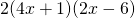Chapter 6: Polynomials

# 6.5 Multiplication of Polynomials

Multiplying monomials is done by multiplying the numbers or coefficients and then adding the exponents on like factors. This is shown in the next example.

Example 6.5.1

Find the following product: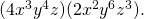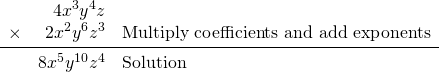Some notes: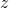has an exponent of 1 when no exponent is written. When adding or subtracting, the exponents will stay the same, but when multiplying (or dividing), the exponents will change.

Next,  consider multiplying a monomial by a polynomial. We have seen this operation before with distributing throughout parentheses. Here, its the exact same process.

Example 6.5.2

Find the following product: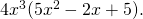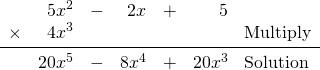Example 6.5.3

Find the following product: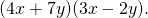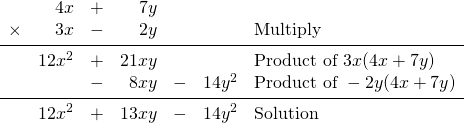Example 6.5.4

Find the following product: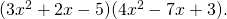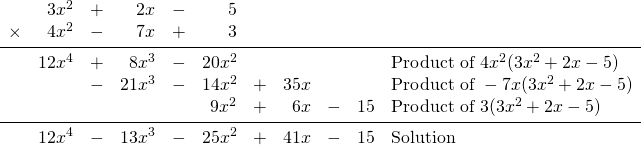Example 6.5.5

Find the following product: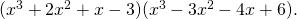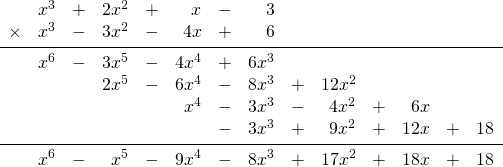As seen in the last two examples, the strategy used is that of foiling, with the only difference being that the answers are organized into columns. This eliminates the need to chase terms scattered all over the page, as they are now grouped.

This is the superior strategy to use when multiplying polynomials.

# Questions

Find each product.

1.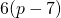2.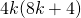3.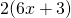4.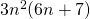5.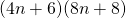6.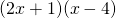7.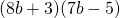8.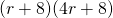9.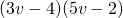10.11.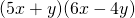12.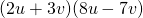13.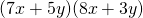14.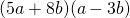15.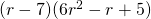16.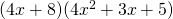17.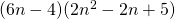18.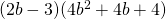19.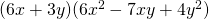20.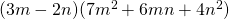21.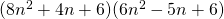22.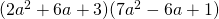23.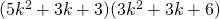24.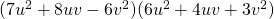25.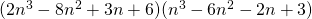26.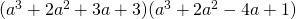27.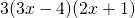28.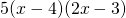29.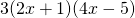30.